# Images of the Experiment

## Others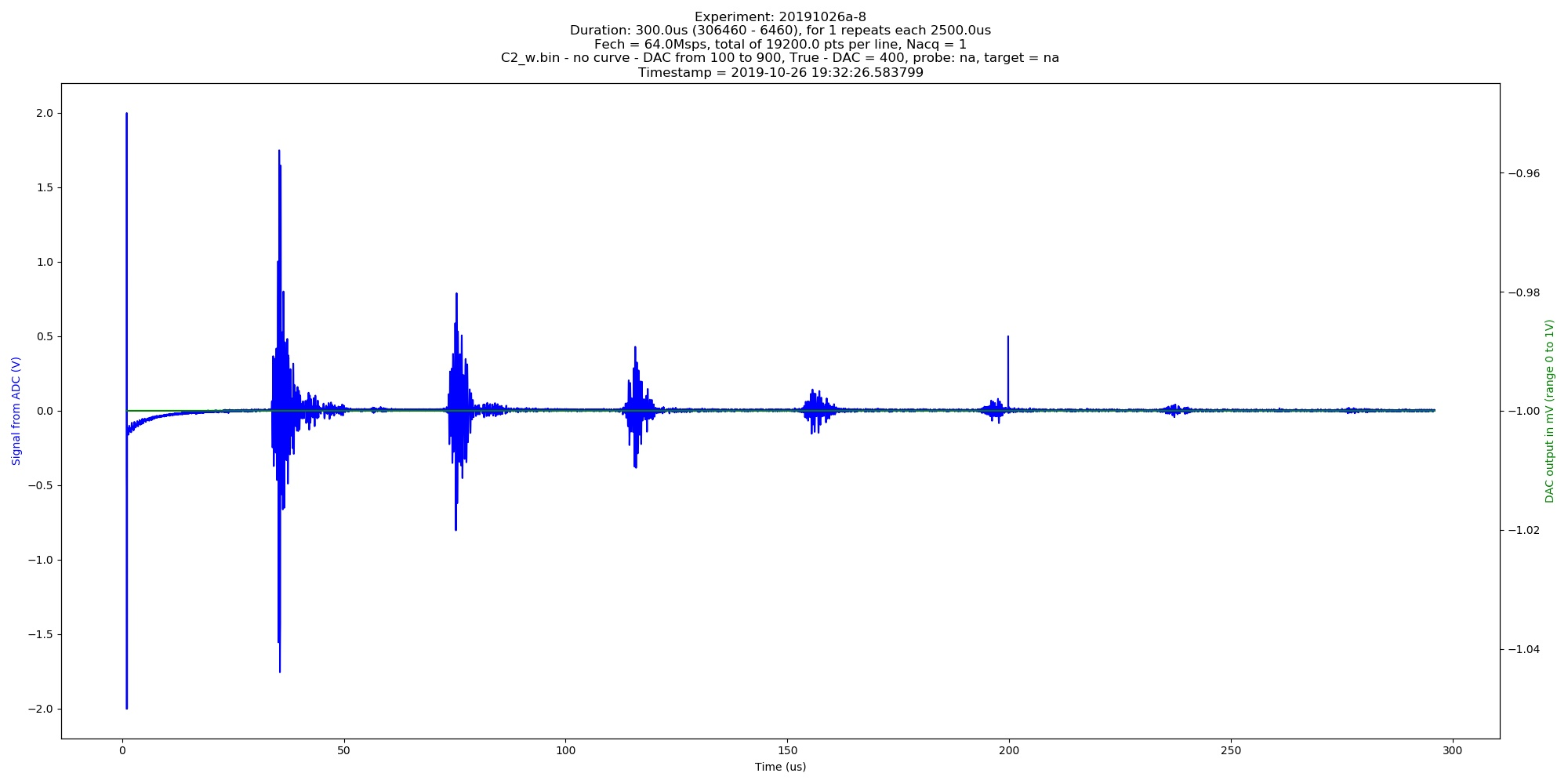Automated image of 20191026a experiment. C2w.bin - no curve - DAC from 100 to 900, True - DAC = 400 (category: _graph).Automated image of 20191026a experiment. Da.bin - no curve - DAC from 100 to 900, True - DAC = 400 (category: graph).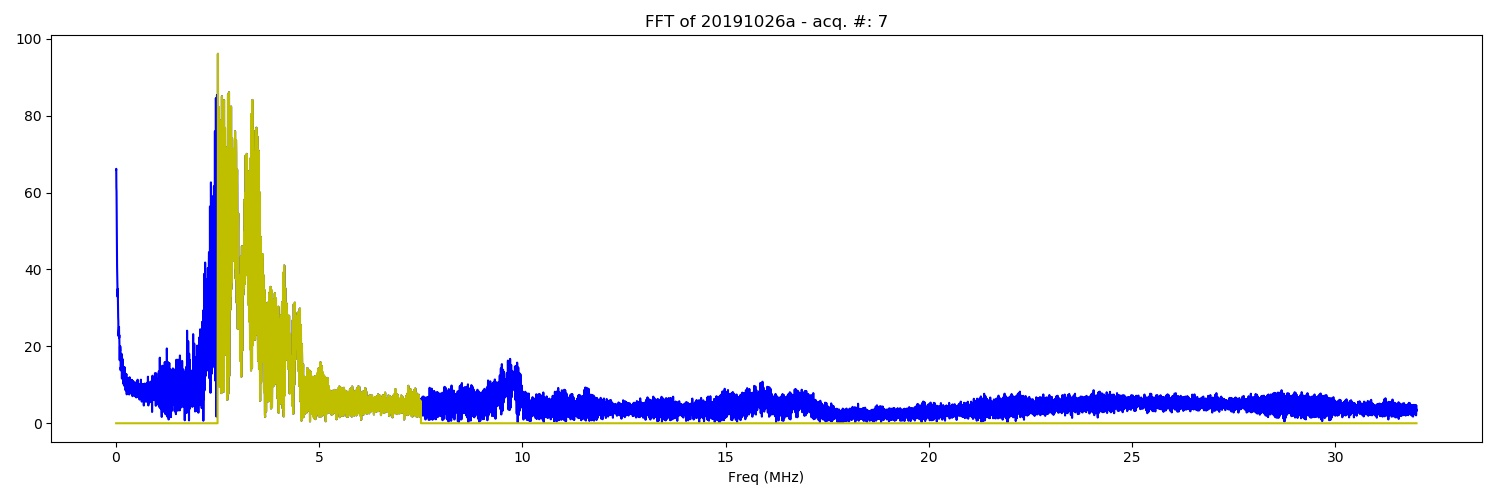FFT of the of 20191026a experiment. C2w.bin - no curve - DAC from 100 to 900, True - DAC = 400 (category: _FFT).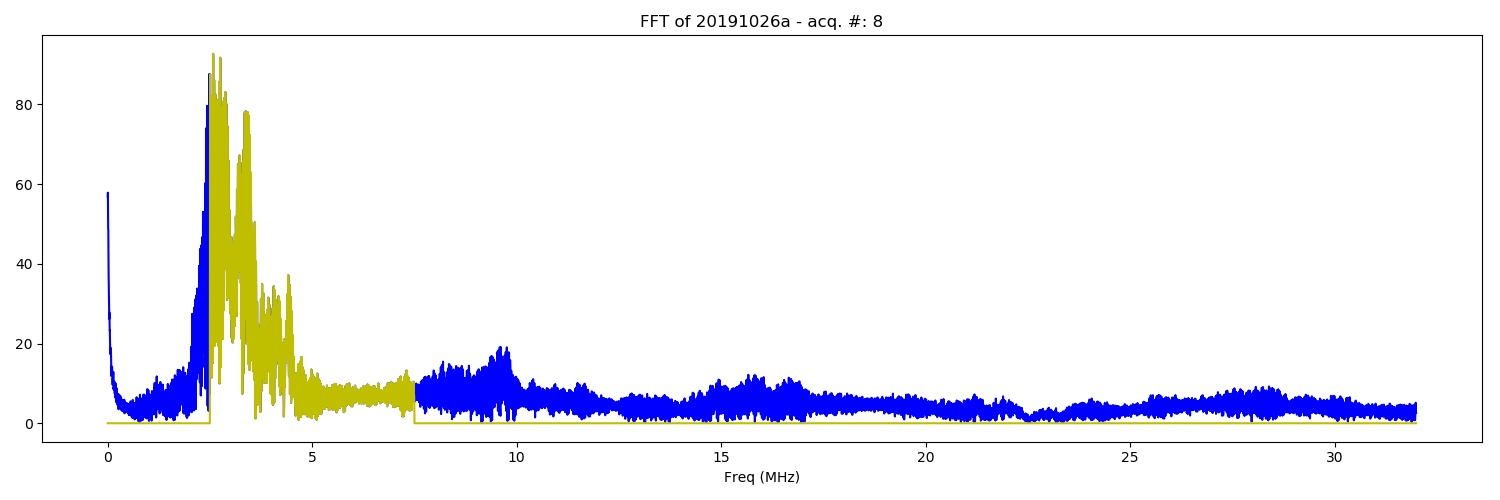FFT of the of 20191026a experiment. C2w.bin - no curve - DAC from 100 to 900, True - DAC = 400 (category: _FFT).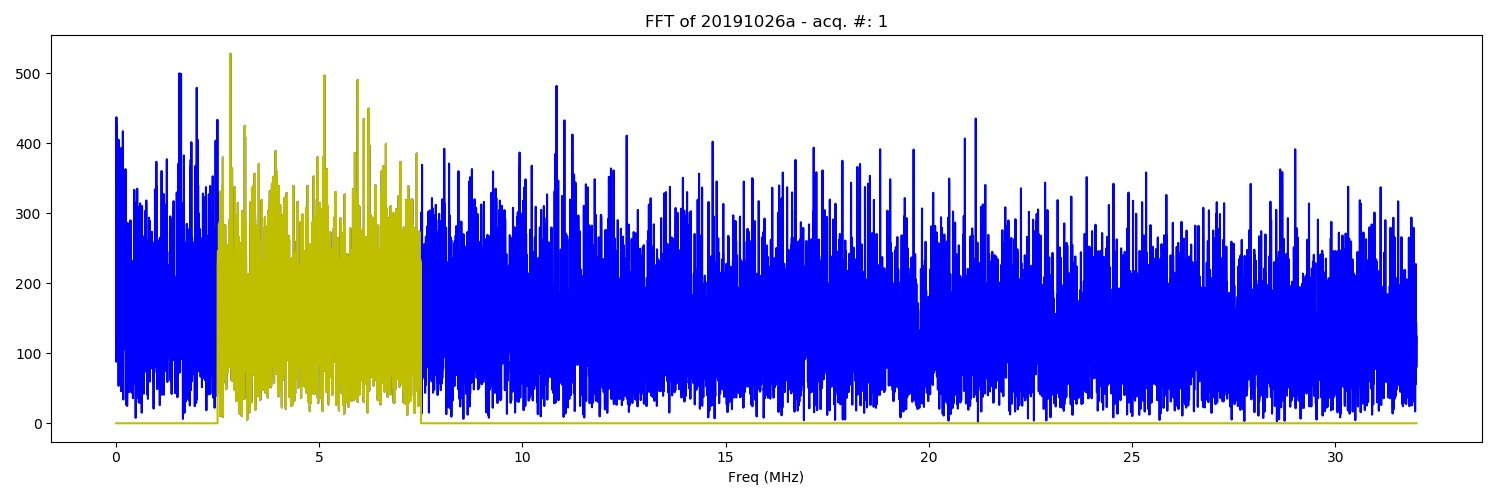FFT of the of 20191026a experiment. Dc.bin - DAC from 100 to 900, True - DAC = 400 (category: _FFT).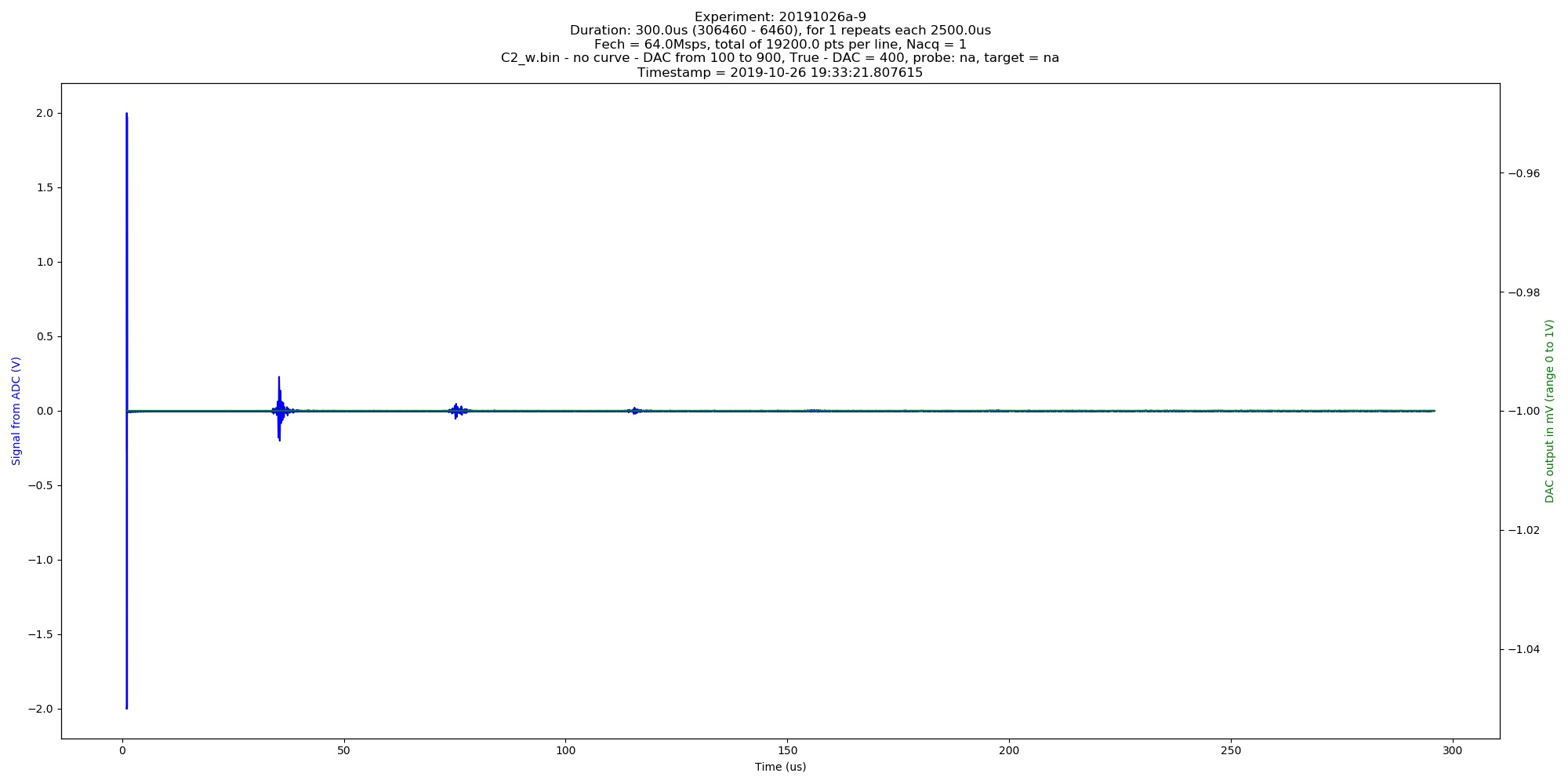Automated image of 20191026a experiment. C2w.bin - no curve - DAC from 100 to 900, True - DAC = 400 (category: _graph).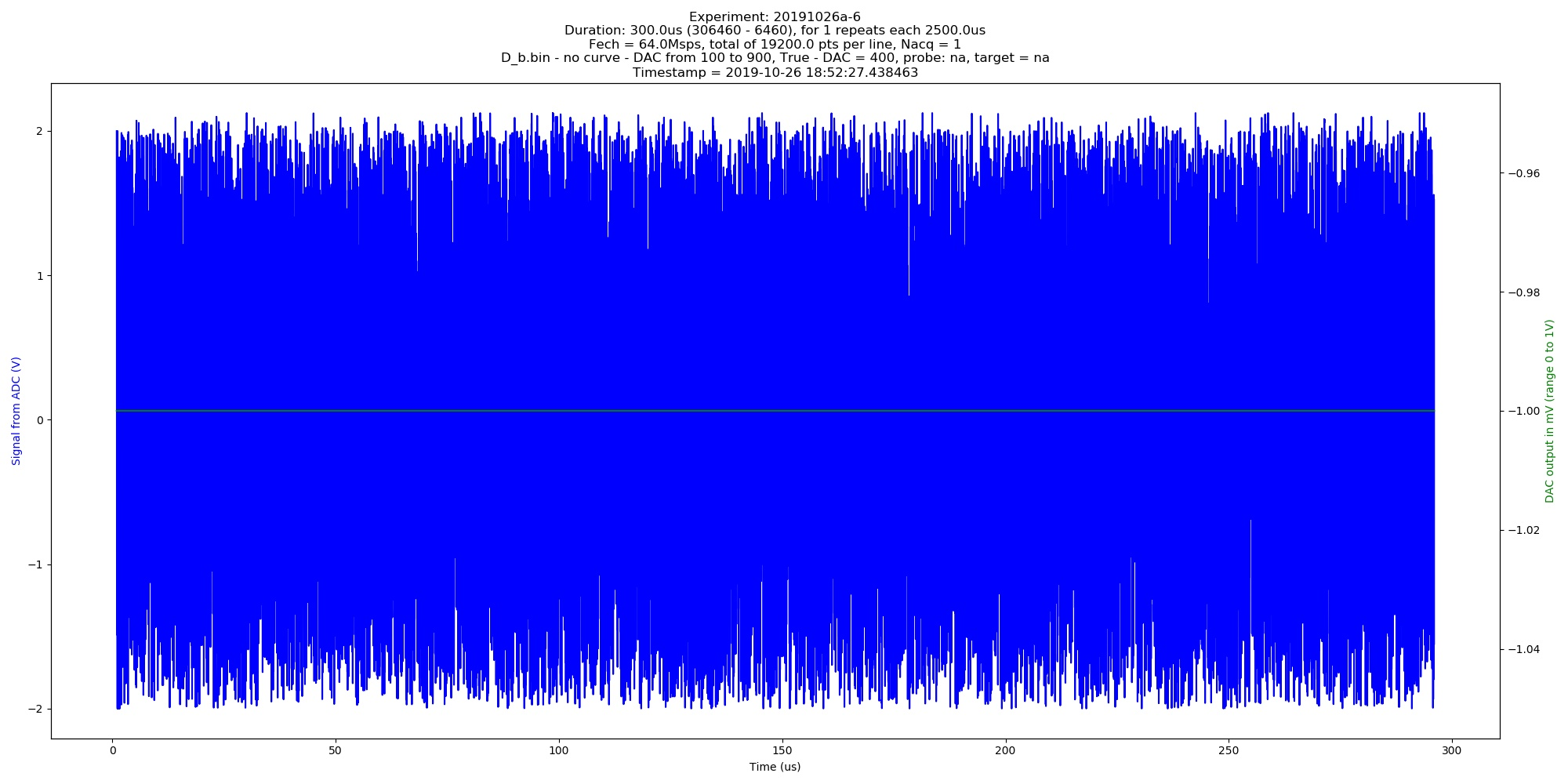Automated image of 20191026a experiment. Db.bin - no curve - DAC from 100 to 900, True - DAC = 400 (category: _graph).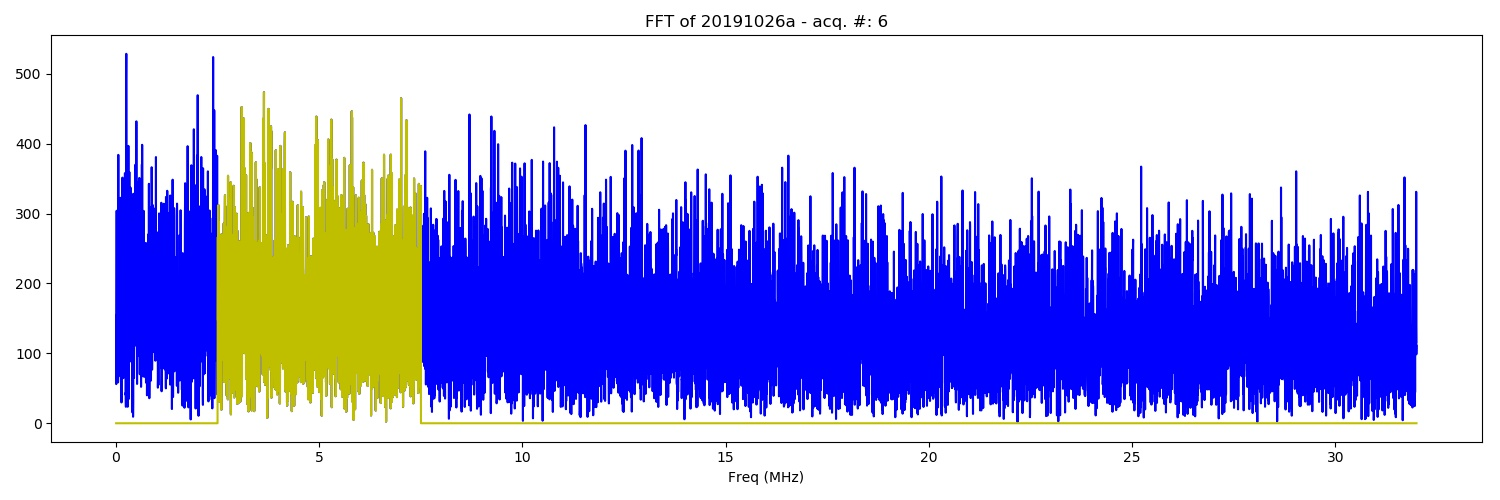FFT of the of 20191026a experiment. Db.bin - no curve - DAC from 100 to 900, True - DAC = 400 (category: _FFT).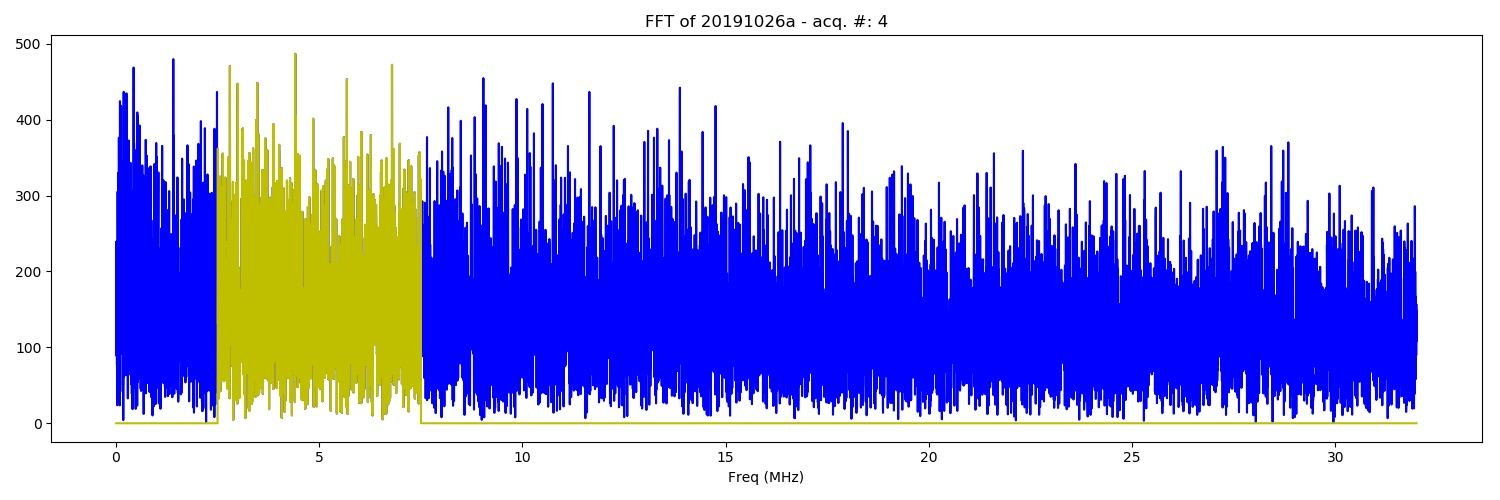FFT of the of 20191026a experiment. Da.bin - DAC from 100 to 900, True - DAC = 400 (category: _FFT).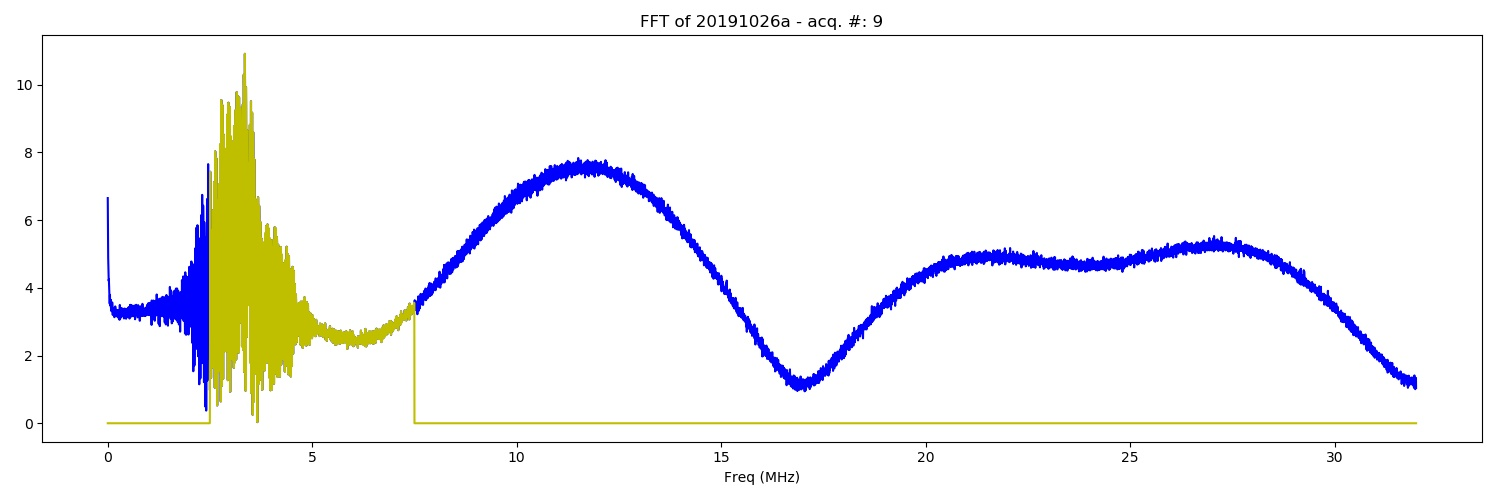FFT of the of 20191026a experiment. C2w.bin - no curve - DAC from 100 to 900, True - DAC = 400 (category: _FFT).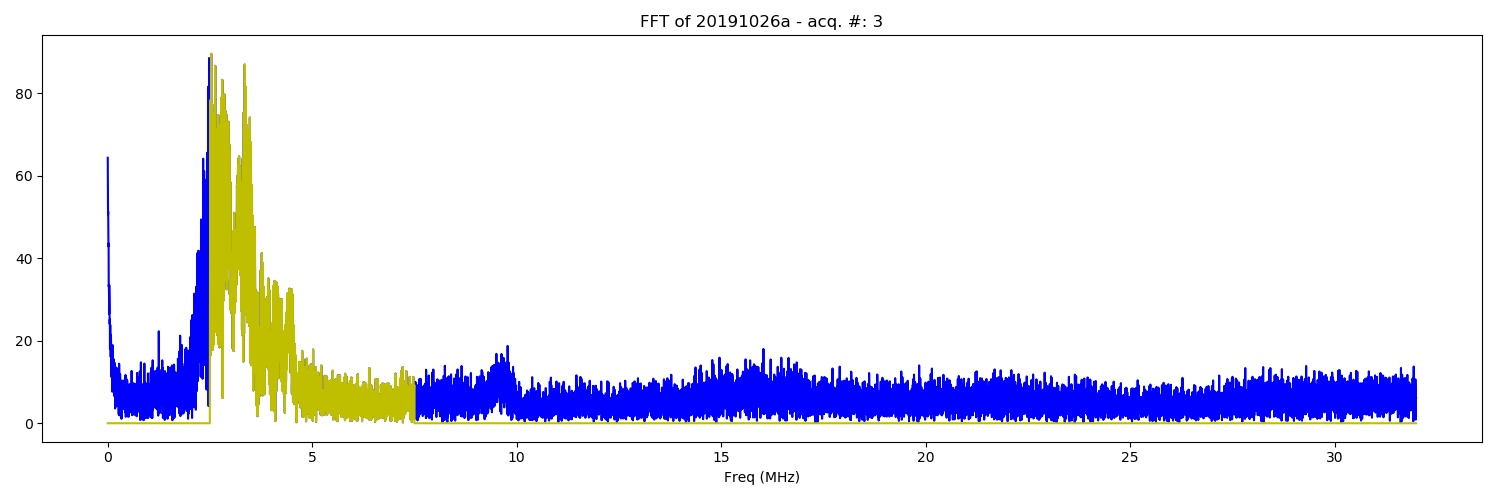FFT of the of 20191026a experiment. C2w.bin - DAC from 100 to 900, True - DAC = 400 (category: _FFT).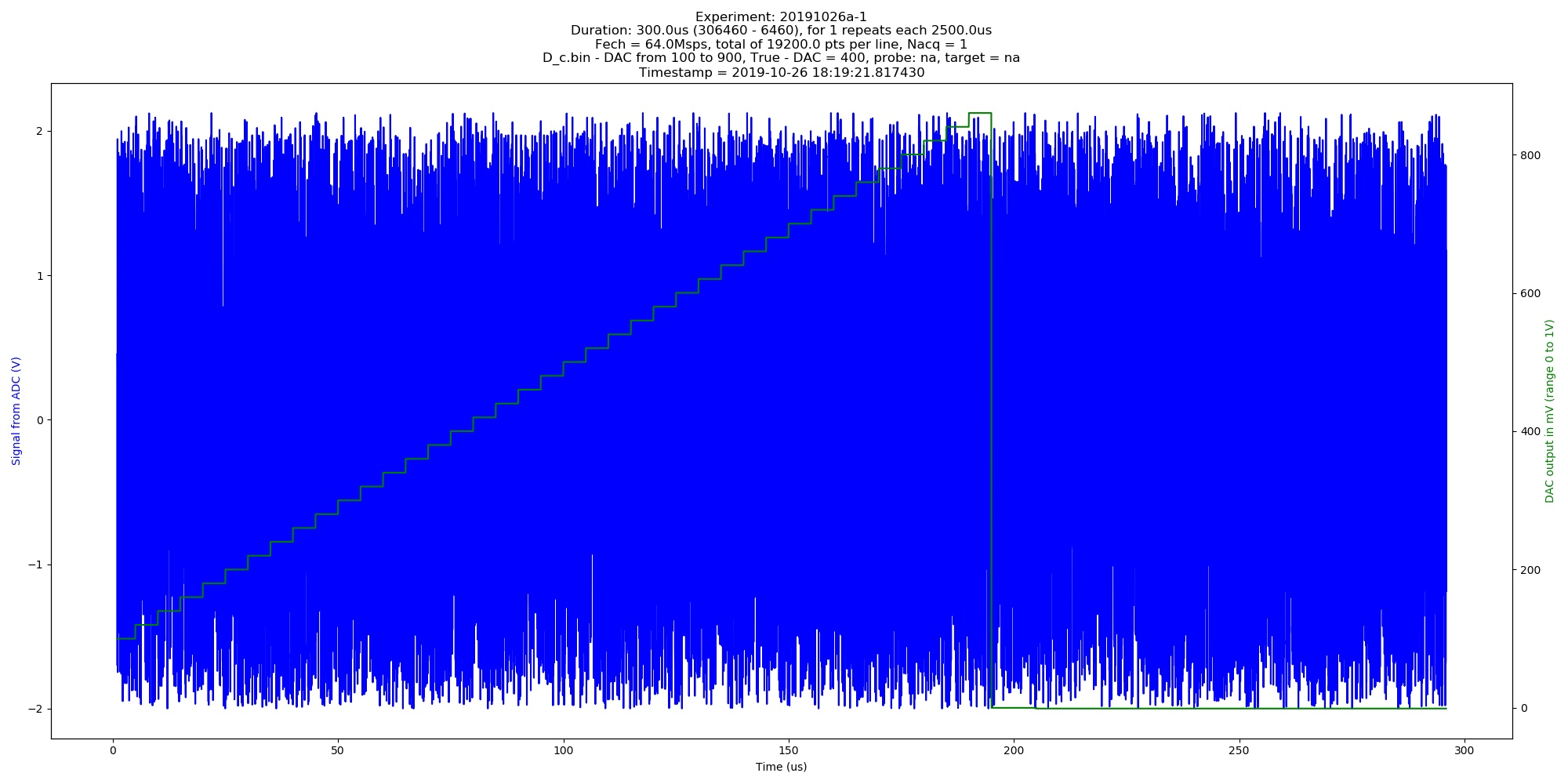Automated image of 20191026a experiment. Dc.bin - DAC from 100 to 900, True - DAC = 400 (category: _graph).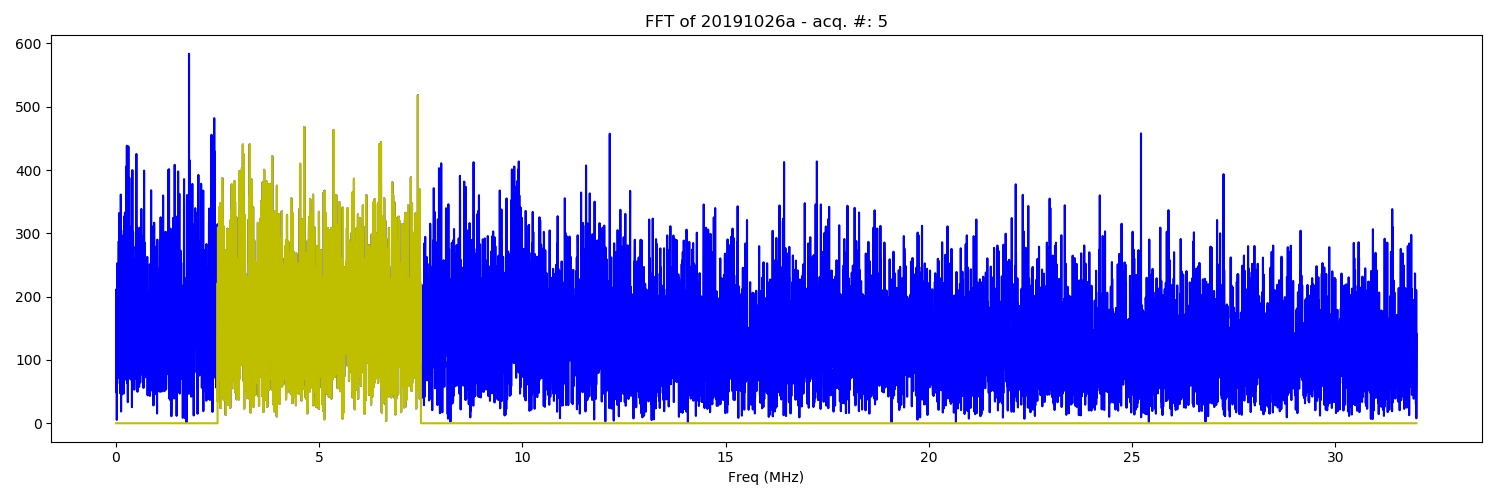FFT of the of 20191026a experiment. Da.bin - no curve - DAC from 100 to 900, True - DAC = 400 (category: _FFT).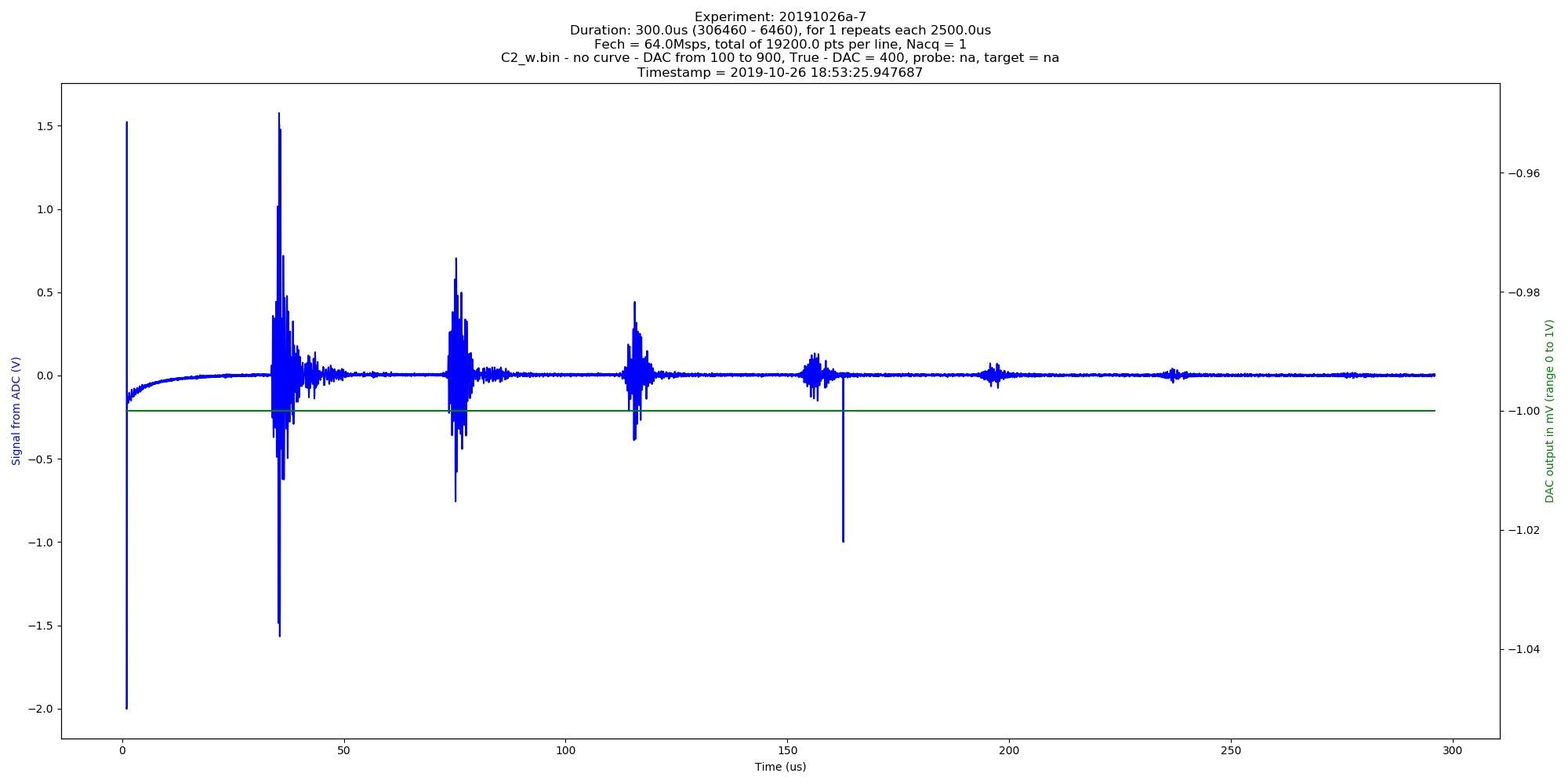Automated image of 20191026a experiment. C2w.bin - no curve - DAC from 100 to 900, True - DAC = 400 (category: _graph).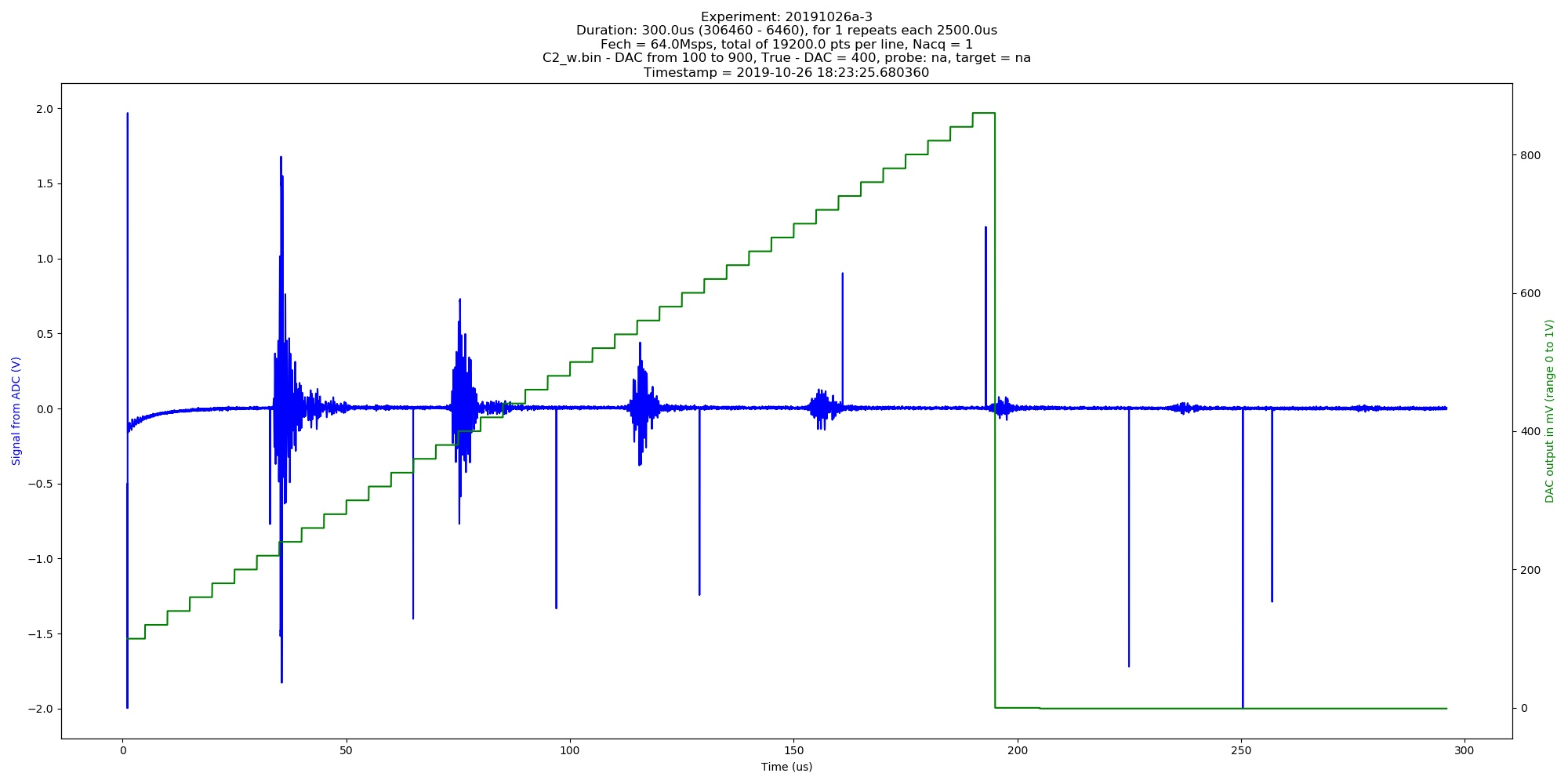Automated image of 20191026a experiment. C2w.bin - DAC from 100 to 900, True - DAC = 400 (category: _graph).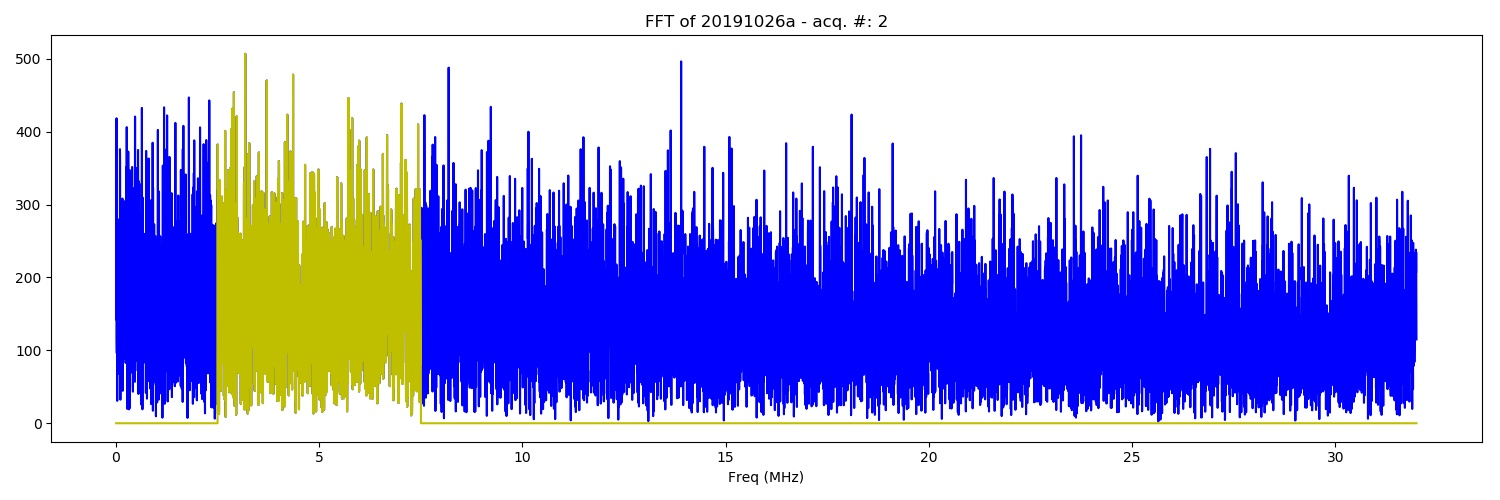FFT of the of 20191026a experiment. Da.bin - DAC from 100 to 900, True - DAC = 400 (category: _FFT).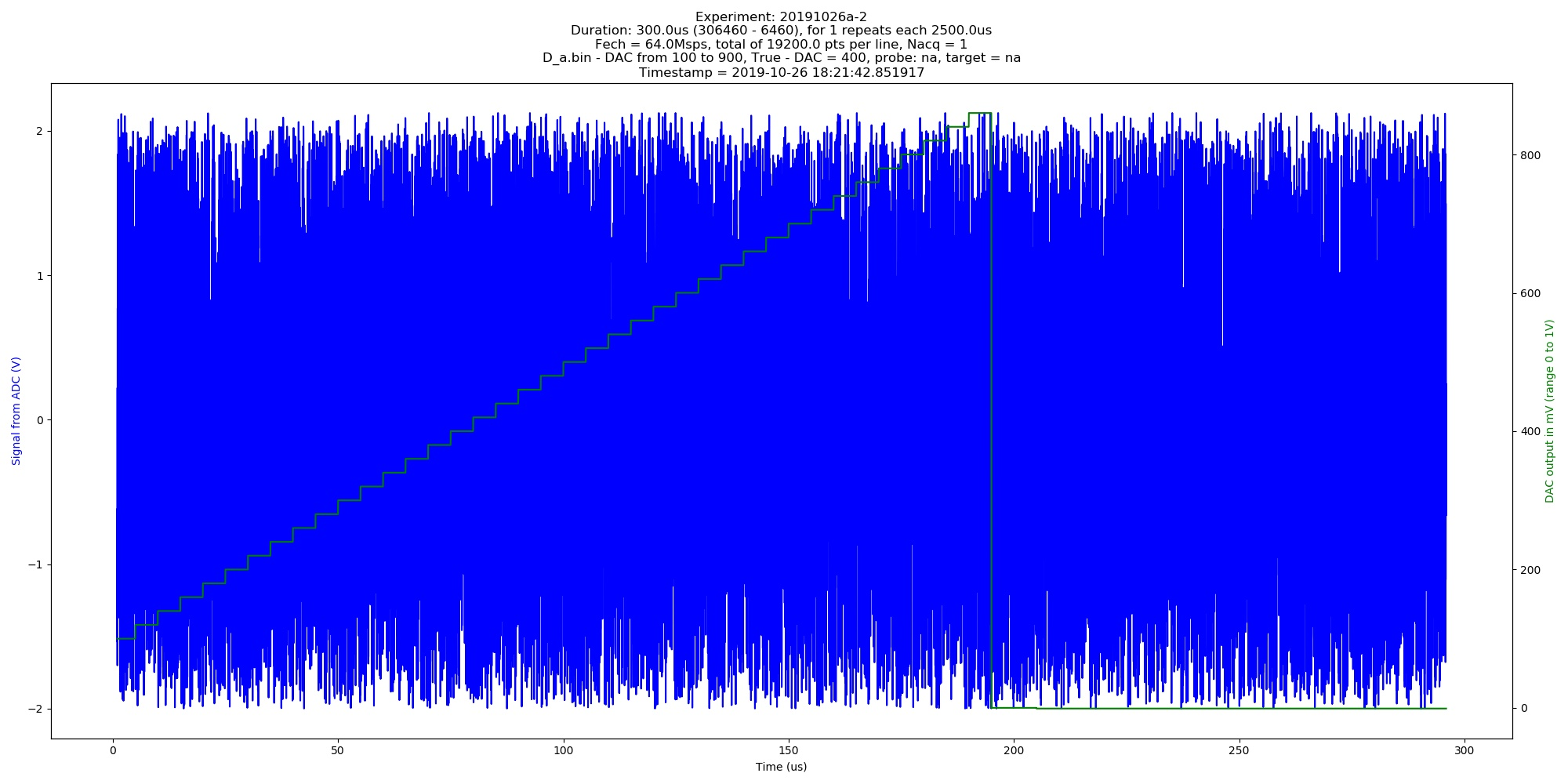Automated image of 20191026a experiment. Da.bin - DAC from 100 to 900, True - DAC = 400 (category: _graph).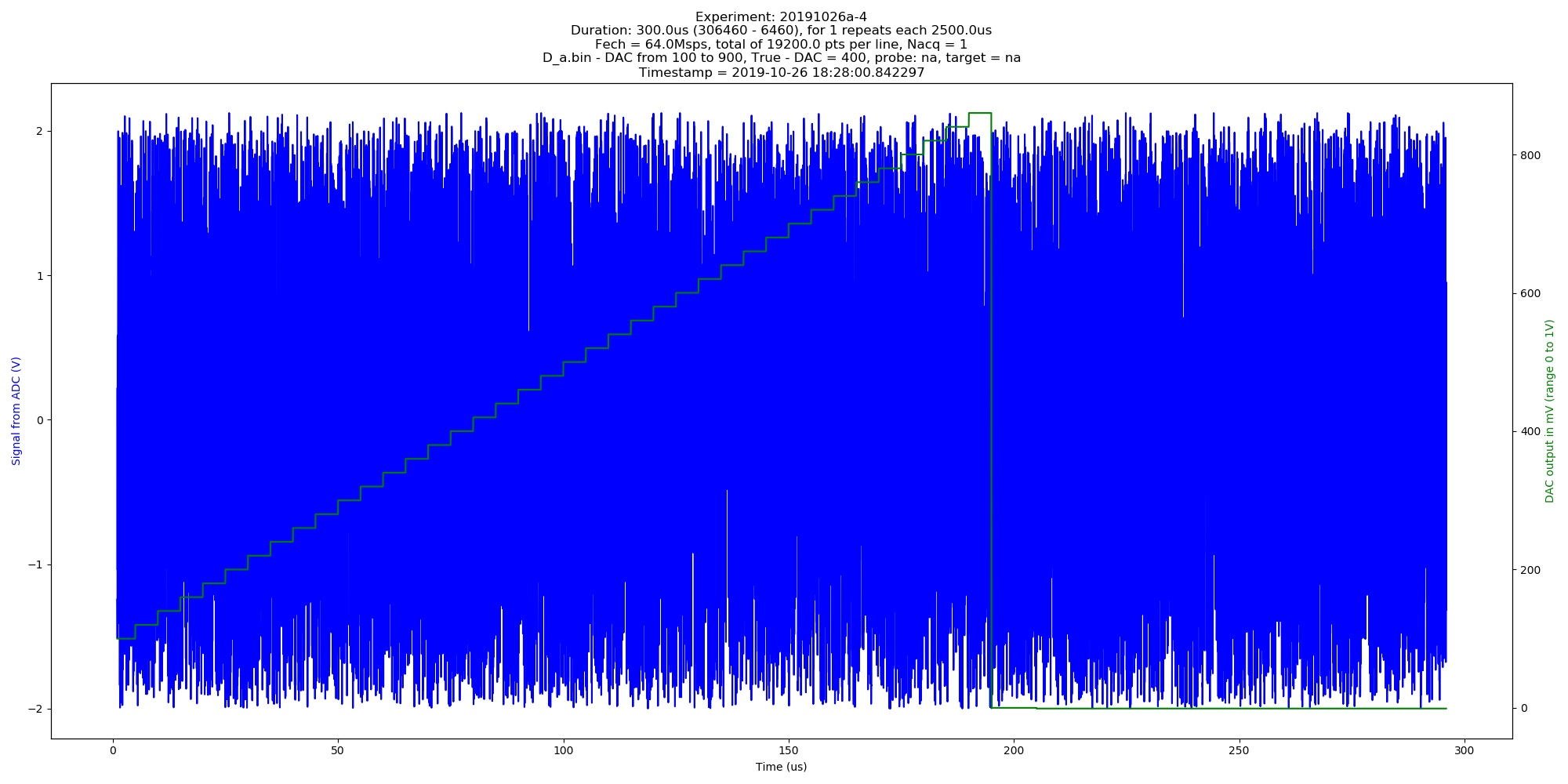Automated image of 20191026a experiment. Da.bin - DAC from 100 to 900, True - DAC = 400 (category: _graph).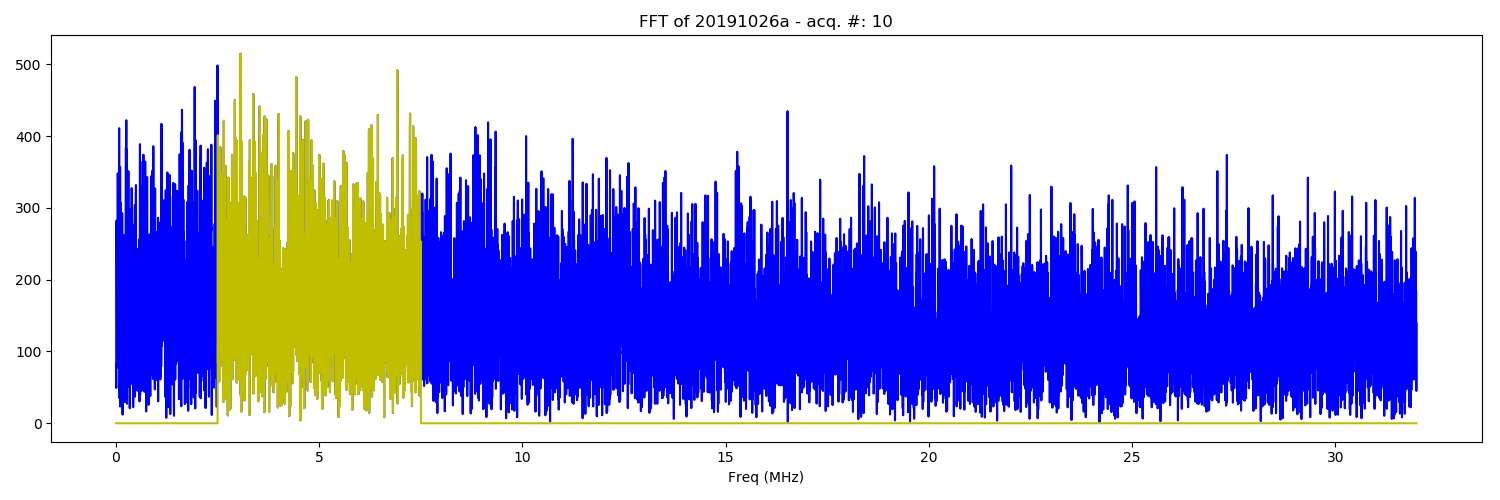FFT of the of 20191026a experiment. Da.bin - no curve - DAC from 100 to 900, True - DAC = 400 (category: FFT).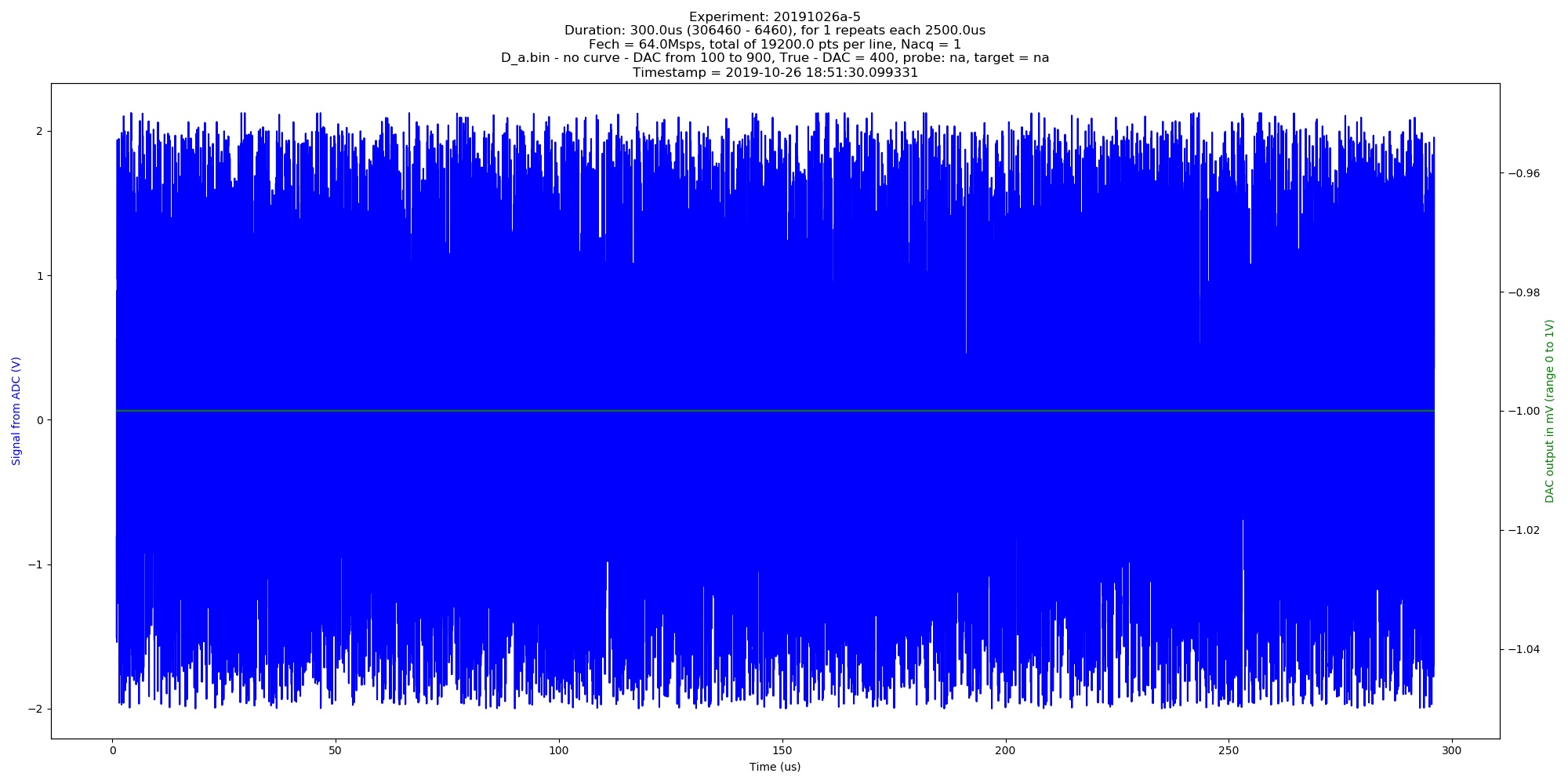Automated image of 20191026a experiment. Da.bin - no curve - DAC from 100 to 900, True - DAC = 400 (category: _graph).